Molecule Types Linear Molecules

# Linear Molecule Perturbation

There is one of these for each perturbation parameter in each state. There are many possible perturbation types, as described below. To see the actual matrix elements used in any particular case, right click on the perturbation and select "Matrix Elements". The resulting expressions will be displayed in the log window, and should be understood as being multiplied by the Value parameter.

## Settings

 Nucleus Index, starting from 1, of the nuclear spin involved in perturbation; 0 (the default) for those not involving a nuclear spin. SymSelect Possible values are all (normal use), e_only (only affect e levels) and f_only (only affect f levels). Was ParitySelect in previous versions. ScalePrev Scale factor with respect to preceding perturbation Op Perturbation type. See below for possible values. If only Npower and/or Srank, Scomp are needed, use Op = Luncouple with n = 0. n Power of operator in perturbation. Npower Power of N (for centrifugal distortion of perturbation). Perturbation operator is ½[N2,Op]+. If RSquaredH is set, N is replaced by R. OmegaSelect Set to all (the default) for normal use; set to a specific value to restrict the perturbation to a specific value of Ω. Note that the current implementation applies the constraint to both connected wavefunctions, so is only useful for ΔΩ = 0 matrix elements. Srank If Srank <= 0: adds a term S±Scomp and changes the power of J or N. See the Op details below. If Srank > 0: spherical tensor operator for S with overall rank Srank and component Scomp. Note that both of these are only implemented for Op = Luncouple, Suncouple and LNuncouple. Versions before 10.033 require Srank < 0 for S±Scomp. Scomp

## Parameters

 Value Size of perturbation; the expressions and operators given below should be understood to be multiplied by this.

The matrix elements are described individually below, but a general comment on specifying the value of the parameter is appropriate. In a Hund’s case (a) basis there are four related matrix elements of any operator: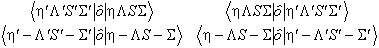PGOPHER will essentially take the one in the top left as the parameter, ordering the matrix such that Λ' > Λ and Λ' > 0 (or Σ' > Σ and Σ' > 0 if Λ' = Λ or S' > S if all the other quantum numbers are equal). The two rows of the matrix can be related using the reflection operator σv:The columns can be related by requiring the Hamiltonian to be symmetric.

## Perturbation Types

LS

Spin orbit coupling, with the Ω dependence from the Wigner-Eckart theorem; see for example Lefebvre-Brion and Field, 2nd Edition Equation 3.4.36: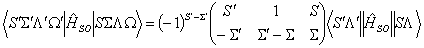The perturbation parameter is the reduced matrix element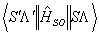arranged so that Λ' > Λ and Λ' > 0 (or S' > S if Λ' = Λ). It is perhaps more normal to quote the value including the 3j symbol, but this is ambiguous when the spin-orbit operator connects more than one component of a pair of states. Some common non-zero values of the 3j symbol are tabulated below.

 S. Σ' S Σ½ -½ ½ ½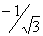½ ½ ½ ½1 0 0 01 1 0 0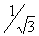1 1 1 11 1 1 0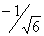Luncouple
J+nL-n+J-nL+n
L uncoupling; Using the default n = 1 this can be used to model the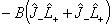term omitted from the rotational operator. The perturbation parameter is the value of the matrix element:for Λ > 0 with the matrix element of L- derived by symmetry
If Srank <= 0 the operator becomes: J+n+ScompS+-ScompL-n+J-n+ScompS--ScompL+n
Suncouple
J+nS-n+J-nS+n S uncoupling. (Note that this term with n = 1 is present in the normal Hamiltonian within a single vibronic state; it only makes sense to add it as a perturbation between states.) It checks for ΔΛ = 0 and |ΔΣ| = n, but does not check for ΔS = 0. S is taken from the Ket. (Note this form is not useful with Srank <= 0).
Homog

Simple homogeneous electrostatic or vibrational perturbation with a ΔΩ = 0 selection rule with no Ω or J dependence.
NS

N.S (n is ignored)
RS

R.S (n is ignored)
LNuncouple
N+nL-n+N-nL+n Similar to Luncouple, but with J replaced by N.
If Srank <= 0 the operator becomes: N+n+ScompS+-ScompL-n+N-n+ScompS--ScompL+n
aL
aI.L
Nuclear Spin Orbit perturbation
e

Nuclear magnetic dipole perturbation
eQq1

## Rotational Hamiltonian expressed as perturbations

The standard rotational Hamiltonian can be expressed entirely in terms of diagonal perturbations, as shown in the table below. The scale factor indicates the factor the standard parameter must be multiplied by to use the given perturbation operator form. This is useful as it allows additional terms to be added, such as higher powers of the centrifugal distortion terms or alternative forms of the lambda doubling to be added. Note that the "Op" is irrelevant if n = 0, but Luncouple should be used in this case.
 Name Operator Op n Npower Srank Scomp Scale Factor Notes B N2 (Luncouple) 0 2 0 0 1 N2 is replaced by R2 if RSquaredH is set D N4 (Luncouple) 0 4 0 0 -1 N4 is replaced by R4 if RSquaredH is set H N6 (Luncouple) 0 6 0 0 1 N6 is replaced by R6 if RSquaredH is set L N8 (Luncouple) 0 8 0 0 1 N8 is replaced by R8 if RSquaredH is set M N10 (Luncouple) 0 10 0 0 1 N10 is replaced by R10 if RSquaredH is set PP N12 (Luncouple) 0 12 0 0 1 N12 is replaced by R12 if RSquaredH is set A see above LS 1 0 0 0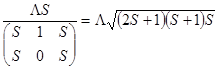which gives the LzSz operator form AD see above LS 1 2 0 0 Scale factor as for A above which gives the ½[N2,LzSz]+ operator form AH see above LS 1 4 0 0 Scale factor as for A above which gives the ½[N4,LzSz]+ operator form AL see above LS 1 6 0 0 Scale factor as for A above which gives the ½[N6,LzSz]+ operator form AM see above LS 1 8 0 0 Scale factor as for A above which gives the ½[N8,LzSz]+ operator form gamma N.S NS 1 0 0 0 1 gammaD ½[N2,N.S]+ NS 1 2 0 0 1 N2 (only) is replaced by R2 if RSquaredH is set gammaH ½[N4,N.S]+ NS 1 4 0 0 1 N4 (only) is replaced by R4 if RSquaredH is set gammaL ½[N6,N.S]+ NS 1 6 0 0 1 N6 (only) is replaced by R6 if RSquaredH is set LambdaSS 6-½(3Sz2-S2) (Luncouple) 0 0 2 0 (8/3)½ LambdaD ½[N2,6-½(3Sz2-S2)]+ (Luncouple) 0 2 2 0 (8/3)½ N2 (only) is replaced by R2 if RSquaredH is set LambdaH ½[N4,6-½(3Sz2-S2)]+ (Luncouple) 0 4 2 0 (8/3)½ N4 (only) is replaced by R4 if RSquaredH is set theta 280-½(35Sz4-20S2Sz2+25Sz2-6S2+3S4) (Luncouple) 0 0 4 0 (35/18)½

### Lambda doubling for Π states

For all of these operators, the N is replaced by R if RSquaredH is set; the N+ and N- operators are not changed.

 Name Operator Op n Npower Srank Scomp Scale Factor o S+2L-2+S-2L+2 LNuncouple 2 0 -1 -2 ½ oD ½[N2,S+2L-2+S-2L+2]+ LNuncouple 2 2 -1 -2 ½ oH ½[N4,S+2L-2+S-2L+2]+ LNuncouple 2 4 -1 -2 ½ oL ½[N6,S+2L-2+S-2L+2]+ LNuncouple 2 6 -1 -2 ½ p N+S+L-2+N-S-L+2 LNuncouple 2 0 -1 -1 -½ pD ½[N2,N+S+L-2+N-S-L+2]+ LNuncouple 2 2 -1 -1 -½ pH ½[N4,N+S+L-2+N-S-L+2]+ LNuncouple 2 4 -1 -1 -½ pL ½[N6,N+S+L-2+N-S-L+2]+ LNuncouple 2 6 -1 -1 -½ q N+2L-2+N-2L+2 LNuncouple 2 0 0 0 ½ qD ½[N2,N+2L-2+N-2L+2]+ LNuncouple 2 2 0 0 ½ qH ½[N4,N+2L-2+N-2L+2]+ LNuncouple 2 4 0 0 ½ qL ½[N6,N+2L-2+N-2L+2]+ LNuncouple 2 6 0 0 ½

An alternative form for these operators, used in some circumstances, can be obtained by replacing LNuncouple with Luncouple. This has the effect of replacing N+ and N- with J+ and J- throughout. For example p + 2q is the determinable constant in some circumstances, and corresponds to the operator ½(J+S+L-2+J-S-L+2). This can be created from the perturbation corresponding to "p" above, with Op = Luncouple, n = 2, Srank = -1 and Scomp = -1.

### Lambda doubling for Δ and higher states

A few lambda doubling operators for Δ states are built into PGOPHER, though note that these use the form involving J rather than N, and the name is not standard for some of them. The form involving N can be obtained by replacing Luncouple with LNuncouple and lambda operators for higher values of Λ can be obtained by setting n = 2Λ. Centrifugal distortion can be added using NPower, as for Π states above.

 Name Operator Op n NPower Srank Scomp Scale Factor Notes o S+4L-4+S-4L+4 Luncouple 4 0 -1 -4 ½ The conventional name would be m oD J+S+3L-4+J-S-3L+4 Luncouple 4 0 -1 -3 -½ The conventional name would be n oH J+2S+2L-4+J-2S-2L+4 Luncouple 4 0 -1 -2 ½ The conventional name would be o p J+3S+L-4+J-3S-L+4 Luncouple 4 0 -1 -1 -½ q J+4L-4+J-4L+4 Luncouple 4 0 0 0 ½